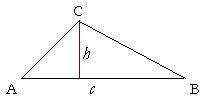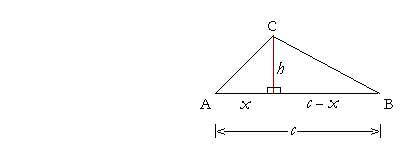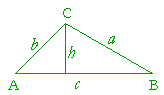2

# Definitions of the Trigonometric Functionsof an Acute Angle

BEFORE DEFINING THE TRIGONOMETRIC FUNCTIONS, we must see how to relate the angles and sides of a right triangle.A right triangle is composed of a right angle, the angle at C, and two acute angles, which are angles less than a right angle.  It is conventional to label the acute angles with Greek letters. We will label the angle at B with the letter θ ("THAY-ta").  And we will label the angle at A with the letter φ ("fie").

As for the sides, the side AB, opposite the right angle, is called the hypotenuse ("hy-POT'n-yoos").  Each acute angle is formed by the hypotenuse and the side adjacent to the angle.  Thus, angle θ is formed by the hypotenuse and side BC.  Angle φ is formed by the hypotenuse and side AC.

With respect to angle θ, though, side AC is its opposite side.  While side BC is the side opposite φ.

The ratios of sides

Any two sides of the triangle will have a ratio -- a relationship -- to one another.  It is possible to form six such ratios:  the ratio of the opposite side to the hypotenuse; the adjacent side to the hypotenuse; and so on. Those six ratios have historical names and abbreviations, with which the student will have to make peace.  They are the following.sine of θ = sin θ = opposite  hypotenuse cosecant of θ = csc θ = hypotenuse  opposite cosine of θ = cos θ = adjacent  hypotenuse secant of θ = sec θ = hypotenuse  adjacent tangent of θ = tan θ = opposite adjacent cotangent of θ = cot θ = adjacentopposite

Notice that each ratio in the right-hand column is the inverse, or the reciprocal, of the ratio in the left-hand column.

The reciprocal of sin θ is csc θ ; and vice-versa.

The reciprocal of cos θ is sec θ.

And the reciprocal of tan θ is cot θ.

Each ratio moreover is a function of the acute angle.  That is, one quantity is a "function" of another if its value depends on the value of the other.  The circumference of a circle is a function of the radius, because the size of the circumference depends on the size of the radius, and when the radius changes, the circumference also will change.  As we will see in the next Topic, the value of each ratio depends only on the value of the acute angle. That is why we say that those ratios are functions of the acute angle.  We call them the trigonometric functions of an acute angle.  All of trigonometry is based on the definitions of those functions.

Problem 1.   Complete the following with either "opposite,"  "adjacent to," or "hypotenuse."

To see the answer, pass your mouse over the colored area.
To cover the answer again, click "Refresh" ("Reload").a) In a right triangle, the side opposite the right angle is called the

a) hypotenuse.

b) CA is called the side opposite angle θ.

c) BC is called the side adjacent to angle θ.

d) AC is called the side adjacent to angle φ.

e) BC is called the side opposite angle φ.

Problem 2.   The sides of a right triangle are in the ratio 3 : 4 : 5, as shown.  Name and evaluate the six trigonometric functions of angle θ.sin θ = 45 csc θ = 54
 cos θ = 35 sec θ = 53
 tan θ = 43 cot θ = 34

Problem 3.    The sides of a right triangle are in the ratio 8 : 15 : 17, as shown.  Name and evaluate the six trigonometric functions of angle φ.sin φ = 1517 csc φ = 1715
 cos φ = 8 17 sec φ = 17 8
 tan φ = 15 8 cot φ = 8 15

Notice that the sides of this triangle satisfy, as they must, the Pythagorean theorem:

 82 + 152 = 172 64 + 225 = 289

Problem 4.   A straight line makes an angle θ with the x-axis.  The valueof which function of θ is equal to its slope?Problem 5.  The height of a triangle.   Every triangle, right-angled or not, will have at least two acute angles.Let them be the base angles at A and B, so that the base will be the side c.  Show that the height h drawn to that base is

 h  = _____c_____cot A + cot B .

Hint:  The height h will cut the entire triangle into two right triangles.Let x be the segment of the base containing the angle A.  Then the remaining segment is the difference between the whole c and that segment:  c − x.

In the right triangle containing the acute angle A,

 xh = cot A,   or   x = h cot A .   .   .  (1)

In the right triangle containing the acute angle B,

 c − x   h = cot B,   or   c − x = h cot B.

On substituting the expression for x from line (1),

c − h cot A = h cot B,

which implies

c = h cot A + h cot B = h(cot A + cot B).

Therefore, on solving for h,

 h  = _____c_____cot A + cot B .

Problem 6.  The area of a triangle.  Prove:  The area of a triangle is equal to one-half the sine of any angle times the product of the two sides that make the angle.Specifically, prove that

Area of triangle ABC = ½ sin A bc = ½ bc sin A.The area of a triangle is equal to one-half the base times the height.  In triangle ABC, let the base be c.  Then

Area = ½ ch.

Now,

sin A = h/b,

so that

h = b sin A.

Therefore in the expression for the Area, replace h with b sin A:

Area = ½ cb sin A.

Next Topic:  Trigonometry of right triangles

Please make a donation to keep TheMathPage online.
Even \$1 will help.